## 1. Overview

In this tutorial, we’ll explore the concept of pattern matching of strings and how we can make it faster. Then, we’ll walk through its implementation in Java.

## 2. Pattern Matching of Strings

### 2.1. Definition

In strings, pattern matching is the process of checking for a given sequence of characters called a pattern in a sequence of characters called a text.

The basic expectations of pattern matching when the pattern is not a regular expression are:

• the match should be exact – not partial
• the result should contain all matches – not just the first match
• the result should contain the position of each match within the text

### 2.2. Searching for a Pattern

Let’s use an example to understand a simple pattern matching problem:

``````Pattern:   NA
Text:      HAVANABANANA
Match1:    ----NA------
Match2:    --------NA--
Match3:    ----------NA``````

We can see that the pattern NA occurs three times in the text. To get this result, we can think of sliding the pattern down the text one character at a time and checking for a match.

However, this is a brute-force approach with time complexity O(p*t) where p is the length of the pattern, and t is the length of text.

Suppose we have more than one pattern to search for. Then, the time complexity also increases linearly as each pattern will need a separate iteration.

### 2.3. Trie Data Structure to Store Patterns

We can improve the search time by storing the patterns in a trie data structure, which is known for its fast retrieval of items.

We know that a trie data structure stores the characters of a string in a tree-like structure. So, for two strings {NA, NAB}, we will get a tree with two paths: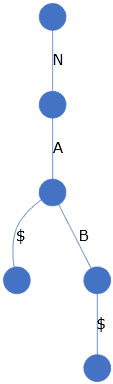Having a trie created makes it possible to slide a group of patterns down the text and check for matches in just one iteration.

Notice that we use the \$ character to indicate the end of the string.

### 2.4. Suffix Trie Data Structure to Store Text

A suffix trie, on the other hand, is a trie data structure constructed using all possible suffixes of a single string.

For the previous example HAVANABANANA, we can construct a suffix trie: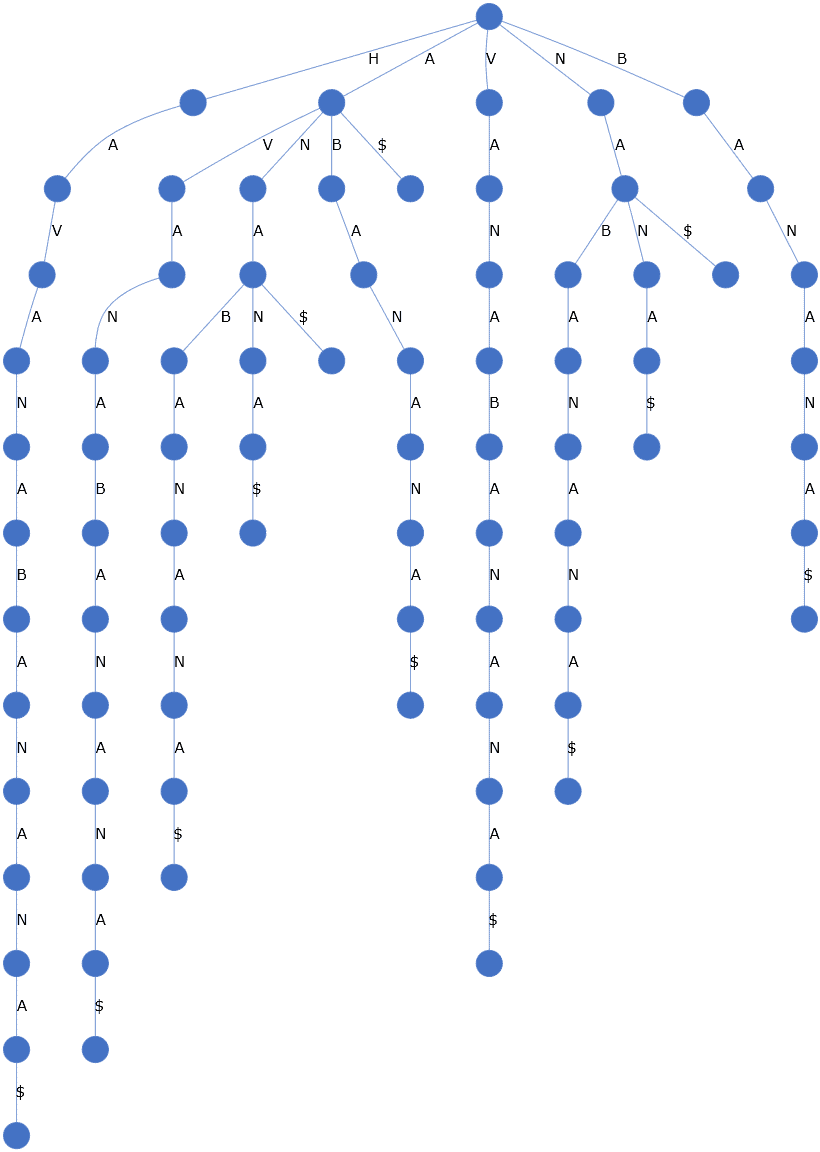Suffix tries are created for the text and are usually done as part of a pre-processing step. After that, searching for patterns can be done quickly by finding a path matching the pattern sequence.

However, a suffix trie is known to consume a lot of space as each character of the string is stored in an edge.

We’ll look at an improved version of the suffix trie in the next section.

## 3. Suffix Tree

A suffix tree is simply a compressed suffix trie. What this means is that, by joining the edges, we can store a group of characters and thereby reduce the storage space significantly.

So, we can create a suffix tree for the same text HAVANABANANA: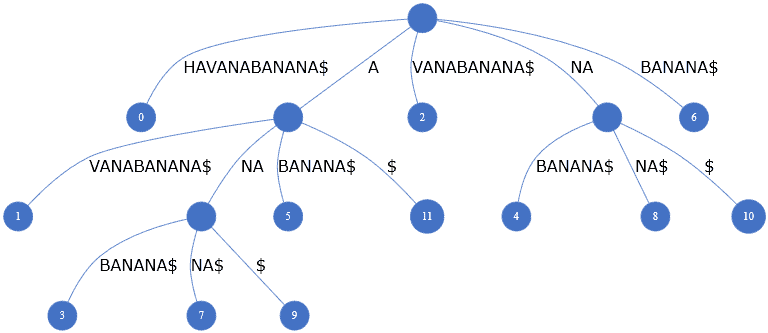Every path starting from the root to the leaf represents a suffix of the string HAVANABANANA.

A suffix tree also stores the position of the suffix in the leaf node. For example, BANANA\$ is a suffix starting from the seventh position. Hence, its value will be six using zero-based numbering. Likewise, A->BANANA\$ is another suffix starting at position five, as we see in the above picture.

So, putting things into perspective, we can see that a pattern match occurs when we’re able to get a path starting from the root node with edges fully matching the given pattern positionally.

If the path ends at a leaf node, we get a suffix match. Otherwise, we get just a substring match. For example, the pattern NA is a suffix of HAVANABANA[NA] and a substring of HAVA[NA]BANANA.

In the next section, we’ll see how to implement this data structure in Java.

## 4. Data Structure

Let’s create a suffix tree data structure. We’ll need two domain classes.

Firstly, we need a class to represent the tree node. It needs to store the tree’s edges and its child nodes. Additionally, when it’s a leaf node, it needs to store the positional value of the suffix.

So, let’s create our Node class:

``````public class Node {
private String text;
private List<Node> children;
private int position;

public Node(String word, int position) {
this.text = word;
this.position = position;
this.children = new ArrayList<>();
}

// getters, setters, toString()
}``````

Secondly, we need a class to represent the tree and store the root node. It also needs to store the full text from which the suffixes are generated.

Consequently, we have a SuffixTree class:

``````public class SuffixTree {
private static final String WORD_TERMINATION = "\$";
private static final int POSITION_UNDEFINED = -1;
private Node root;
private String fullText;

public SuffixTree(String text) {
root = new Node("", POSITION_UNDEFINED);
fullText = text;
}
}``````

## 5. Helper Methods for Adding Data

Before we write our core logic to store data, let’s add a few helper methods. These will prove useful later.

Let’s modify our SuffixTree class to add some methods needed for constructing the tree.

### 5.1. Adding a Child Node

Firstly, let’s have a method addChildNode to add a new child node to any given parent node:

``````private void addChildNode(Node parentNode, String text, int index) {
}``````

### 5.2. Finding Longest Common Prefix of Two Strings

Secondly, we’ll write a simple utility method getLongestCommonPrefix to find the longest common prefix of two strings:

``````private String getLongestCommonPrefix(String str1, String str2) {
int compareLength = Math.min(str1.length(), str2.length());
for (int i = 0; i < compareLength; i++) {
if (str1.charAt(i) != str2.charAt(i)) {
return str1.substring(0, i);
}
}
return str1.substring(0, compareLength);
}``````

### 5.3. Splitting a Node

Thirdly, let’s have a method to carve out a child node from a given parent. In this process, the parent node’s text value will get truncated, and the right-truncated string becomes the text value of the child node. Additionally, the children of the parent will get transferred to the child node.

We can see from the picture below that ANA gets split to A->NA. Afterward, the new suffix ABANANA\$ can be added as A->BANANA\$: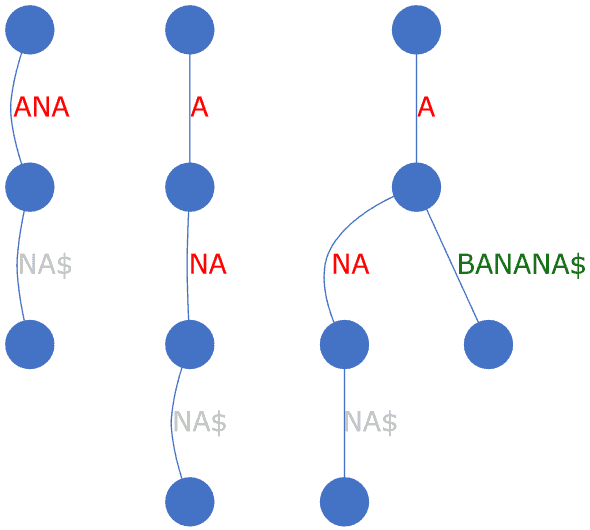In short, this is a convenience method that will come in handy when inserting a new node:

``````private void splitNodeToParentAndChild(Node parentNode, String parentNewText, String childNewText) {
Node childNode = new Node(childNewText, parentNode.getPosition());

if (parentNode.getChildren().size() > 0) {
while (parentNode.getChildren().size() > 0) {
childNode.getChildren()
}
}

parentNode.setText(parentNewText);
parentNode.setPosition(POSITION_UNDEFINED);
}``````

## 6. Helper Method for Traversal

Let’s now create the logic to traverse the tree. We’ll use this method for both constructing the tree and searching for patterns.

### 6.1. Partial Match vs. Full Match

First, let’s understand the concept of a partial match and a full match by considering a tree populated with a few suffixes: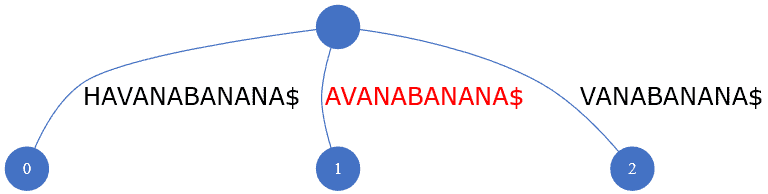To add a new suffix ANABANANA\$, we check if any node exists that can be modified or extended to accommodate the new value. For this, we compare the new text with all the nodes and find that the existing node [A]VANABANANA\$ matches at first character. So, this is the node we need to modify, and this match can be called a partial match.

On the other hand, let’s consider that we’re searching for the pattern VANE on the same tree. We know that it partially matches with [VAN]ABANANA\$ on the first three characters. If all the four characters had matched, we could call it a full match. For pattern search, a complete match is necessary.

So to summarize, we’ll use a partial match when constructing the tree and a full match when searching for patterns. We’ll use a flag isAllowPartialMatch to indicate the kind of match we need in each case.

### 6.2. Traversing the Tree

Now, let’s write our logic to traverse the tree as long as we’re able to match a given pattern positionally:

``````List<Node> getAllNodesInTraversePath(String pattern, Node startNode, boolean isAllowPartialMatch) {
// ...
}``````

We’ll call this recursively and return a list of all the nodes we find in our path.

We start by comparing the first character of the pattern text with the node text:

``````if (pattern.charAt(0) == nodeText.charAt(0)) {
// logic to handle remaining characters
}
``````

For a partial match, if the pattern is shorter or equal in length to the node text, we add the current node to our nodes list and stop here:

``````if (isAllowPartialMatch && pattern.length() <= nodeText.length()) {
return nodes;
}
``````

Then we compare the remaining characters of this node text with that of the pattern. If the pattern has a positional mismatch with the node text, we stop here. The current node is included in nodes list only for a partial match:

``````int compareLength = Math.min(nodeText.length(), pattern.length());
for (int j = 1; j < compareLength; j++) {
if (pattern.charAt(j) != nodeText.charAt(j)) {
if (isAllowPartialMatch) {
}
return nodes;
}
}
``````

If the pattern matched the node text, we add the current node to our nodes list:

``nodes.add(currentNode);``

But if the pattern has more characters than the node text, we need to check the child nodes. For this, we make a recursive call passing the currentNode as the starting node and remaining portion of the pattern as the new pattern. The list of nodes returned from this call is appended to our nodes list if it’s not empty. In case it’s empty for a full match scenario, it means there was a mismatch, so to indicate this, we add a null item. And we return the nodes:

``````if (pattern.length() > compareLength) {
List nodes2 = getAllNodesInTraversePath(pattern.substring(compareLength), currentNode,
isAllowPartialMatch);
if (nodes2.size() > 0) {
} else if (!isAllowPartialMatch) {
}
}
return nodes;``````

Putting all this together, let’s create getAllNodesInTraversePath:

``````private List<Node> getAllNodesInTraversePath(String pattern, Node startNode, boolean isAllowPartialMatch) {
List<Node> nodes = new ArrayList<>();
for (int i = 0; i < startNode.getChildren().size(); i++) {
Node currentNode = startNode.getChildren().get(i);
String nodeText = currentNode.getText();
if (pattern.charAt(0) == nodeText.charAt(0)) {
if (isAllowPartialMatch && pattern.length() <= nodeText.length()) {
return nodes;
}

int compareLength = Math.min(nodeText.length(), pattern.length());
for (int j = 1; j < compareLength; j++) {
if (pattern.charAt(j) != nodeText.charAt(j)) {
if (isAllowPartialMatch) {
}
return nodes;
}
}

if (pattern.length() > compareLength) {
List<Node> nodes2 = getAllNodesInTraversePath(pattern.substring(compareLength),
currentNode, isAllowPartialMatch);
if (nodes2.size() > 0) {
} else if (!isAllowPartialMatch) {
}
}
return nodes;
}
}
return nodes;
}``````

## 7. Algorithm

### 7.1. Storing Data

We can now write our logic to store data. Let’s start by defining a new method addSuffix on the SuffixTree class:

``````private void addSuffix(String suffix, int position) {
// ...
}``````

The caller will provide the position of the suffix.

Next, let’s write the logic to handle the suffix. First, we need to check if a path exists matching the suffix partially at least by calling our helper method getAllNodesInTraversePath with isAllowPartialMatch set as true. If no path exists, we can add our suffix as a child to the root:

``````List<Node> nodes = getAllNodesInTraversePath(pattern, root, true);
if (nodes.size() == 0) {
}``````

However, if a path exists, it means we need to modify an existing node. This node will be the last one in the nodes list. We also need to figure out what should be the new text for this existing node. If the nodes list has only one item, then we use the suffix. Otherwise, we exclude the common prefix up to the last node from the suffix to get the newText:

``````Node lastNode = nodes.remove(nodes.size() - 1);
String newText = suffix;
if (nodes.size() > 0) {
String existingSuffixUptoLastNode = nodes.stream()
.map(a -> a.getText())
.reduce("", String::concat);
newText = newText.substring(existingSuffixUptoLastNode.length());
}``````

For modifying the existing node, let’s create a new method extendNode, which we’ll call from where we left off in addSuffix method. This method has two key responsibilities. One is to break up an existing node to parent and child, and the other is to add a child to the newly created parent node. We break up the parent node only to make it a common node for all its child nodes. So, our new method is ready:

``````private void extendNode(Node node, String newText, int position) {
String currentText = node.getText();
String commonPrefix = getLongestCommonPrefix(currentText, newText);

if (commonPrefix != currentText) {
String parentText = currentText.substring(0, commonPrefix.length());
String childText = currentText.substring(commonPrefix.length());
splitNodeToParentAndChild(node, parentText, childText);
}

String remainingText = newText.substring(commonPrefix.length());
}``````

We can now come back to our method for adding a suffix, which now has all the logic in place:

``````private void addSuffix(String suffix, int position) {
List<Node> nodes = getAllNodesInTraversePath(suffix, root, true);
if (nodes.size() == 0) {
} else {
Node lastNode = nodes.remove(nodes.size() - 1);
String newText = suffix;
if (nodes.size() > 0) {
String existingSuffixUptoLastNode = nodes.stream()
.map(a -> a.getText())
.reduce("", String::concat);
newText = newText.substring(existingSuffixUptoLastNode.length());
}
extendNode(lastNode, newText, position);
}
}``````

Finally, let’s modify our SuffixTree constructor to generate the suffixes and call our previous method addSuffix to add them iteratively to our data structure:

``````public void SuffixTree(String text) {
root = new Node("", POSITION_UNDEFINED);
for (int i = 0; i < text.length(); i++) {
}
fullText = text;
}``````

### 7.2. Searching Data

Having defined our suffix tree structure to store data, we can now write the logic for performing our search.

We begin by adding a new method searchText on the SuffixTree class, taking in the pattern to search as an input:

``````public List<String> searchText(String pattern) {
// ...
}``````

Next, to check if the pattern exists in our suffix tree, we call our helper method getAllNodesInTraversePath with the flag set for exact matches only, unlike during the adding of data when we allowed partial matches:

``List<Node> nodes = getAllNodesInTraversePath(pattern, root, false);``

We then get the list of nodes that match our pattern. The last node in the list indicates the node up to which the pattern matched exactly. So, our next step will be to get all the leaf nodes originating from this last matching node and get the positions stored in these leaf nodes.

Let’s create a separate method getPositions to do this. We’ll check if the given node stores the final portion of a suffix to decide if its position value needs to be returned. And, we’ll do this recursively for every child of the given node:

``````private List<Integer> getPositions(Node node) {
List<Integer> positions = new ArrayList<>();
if (node.getText().endsWith(WORD_TERMINATION)) {
}
for (int i = 0; i < node.getChildren().size(); i++) {
}
return positions;
}``````

Once we have the set of positions, the next step is to use it to mark the patterns on the text we stored in our suffix tree. The position value indicates where the suffix starts, and the length of the pattern indicates how many characters to offset from the starting point. Applying this logic, let’s create a simple utility method:

``````private String markPatternInText(Integer startPosition, String pattern) {
String matchingTextLHS = fullText.substring(0, startPosition);
String matchingText = fullText.substring(startPosition, startPosition + pattern.length());
String matchingTextRHS = fullText.substring(startPosition + pattern.length());
return matchingTextLHS + "[" + matchingText + "]" + matchingTextRHS;
}``````

Now, we have our supporting methods ready. Therefore, we can add them to our search method and complete the logic:

``````public List<String> searchText(String pattern) {
List<String> result = new ArrayList<>();
List<Node> nodes = getAllNodesInTraversePath(pattern, root, false);

if (nodes.size() > 0) {
Node lastNode = nodes.get(nodes.size() - 1);
if (lastNode != null) {
List<Integer> positions = getPositions(lastNode);
positions = positions.stream()
.sorted()
.collect(Collectors.toList());
}
}
return result;
}``````

## 8. Testing

Now that we have our algorithm in place, let’s test it.

First, let’s store a text in our SuffixTree:

``````SuffixTree suffixTree = new SuffixTree("havanabanana");
``````

Next, let’s search for a valid pattern a:

``````List<String> matches = suffixTree.searchText("a");
matches.stream().forEach(m -> LOGGER.debug(m));``````

Running the code gives us six matches as expected:

``````h[a]vanabanana
hav[a]nabanana
havan[a]banana
havanab[a]nana
havanaban[a]na
havanabanan[a]``````

Next, let’s search for another valid pattern nab:

``````List<String> matches = suffixTree.searchText("nab");
matches.stream().forEach(m -> LOGGER.debug(m));
``````

Running the code gives us only one match as expected:

``hava[nab]anana``

Finally, let’s search for an invalid pattern nag:

``````List<String> matches = suffixTree.searchText("nag");
matches.stream().forEach(m -> LOGGER.debug(m));``````

Running the code gives us no results. We see that matches have to be exact and not partial.

Thus, our pattern search algorithm has been able to satisfy all the expectations we laid out at the beginning of this tutorial.

## 9. Time Complexity

When constructing the suffix tree for a given text of length t, the time complexity is O(t).

Then, for searching a pattern of length p, the time complexity is O(p). Recollect that for a brute-force search, it was O(p*t).  Thus, pattern searching becomes faster after pre-processing of the text.

## 10. Conclusion

In this article, we first understood the concepts of three data structures – trie, suffix trie, and suffix tree. We then saw how a suffix tree could be used to compactly store suffixes.

Later, we saw how to use a suffix tree to store data and perform a pattern search.

As always, the source code with tests is available over on GitHub.

### Get started with Spring and Spring Boot, through the Learn Spring course:

>> CHECK OUT THE COURSE